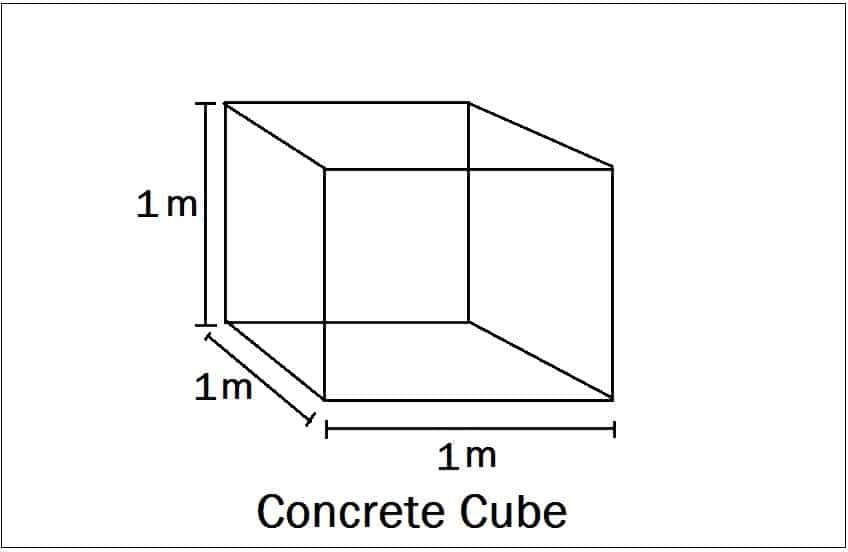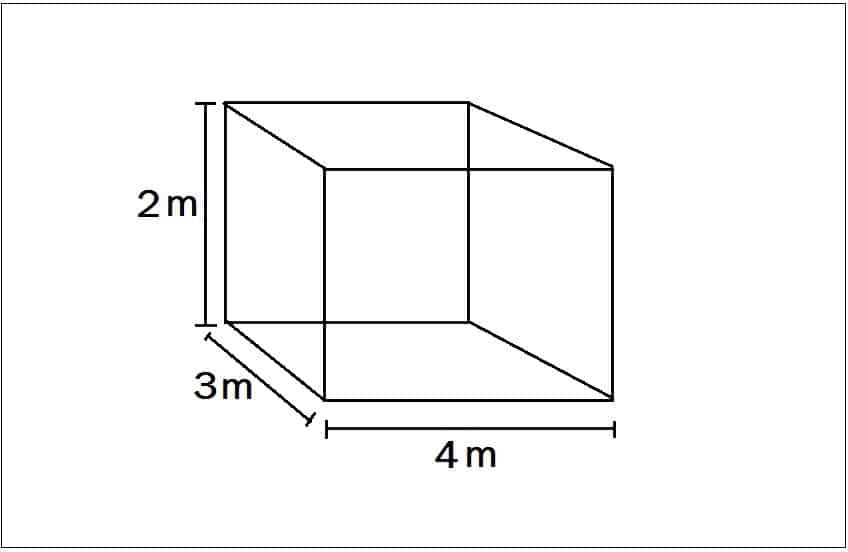# What Is 1.54 In Concrete Calculation

## What Is 1.54 In Concrete Calculation?

In concrete calculation, we always multiply Wet volume with 1.54 to get dry volume. But do you know what is 1.54 or where this 1.54 came from? If You don’t know, no problem. In this article, I will explain what is 1.54 while calculating the quantity of cement, sand, aggregates for concrete.

let us take a concrete cube.The length of  the concrete cube = 1 m

The width of the concrete cube = 1 m

The height of the concrete cube = 1 m

Volume of concrete cube = length x Width x Height = 1 x 1 x 1 = 1 m³ (Wet volume)

When we convert this wet volume into dry volume, the volume is increased by 54% of wet volume.

∴ Dry volume = Wet volume + 54% of Wet volume

= 1 + (54/100) x 1 =1 + 0.54 = 1.54

To convert the wet volume of 1 m³ concrete into dry volume = 1.54

To convert the wet volume of “n” m³ concrete into dry volume = 1.54 x n

Where n = Wet volume of concrete.

Example:The length of  the concrete cube = 4 m

The width of the concrete cube = 3 m

The height of the concrete cube = 2 m

Wet volume = 4 x 3 x 2 = 24 m³

∴ Dry volume = ( Wet volume + 54% of wet volume)

= 24 + {(54/100) x 24}

= 36.96 m³

Note:

Always remember in the concrete calculation, we get wet volume first, to convert wet volume into dry volume, multiply wet volume with 1.54.

### 8 thoughts on “What Is 1.54 In Concrete Calculation”

1. How did the volume of dry concrete come to 54%?

2. Could you please let me know the Wet mix macadam cslculation

3. People become highly benefited in socity.thank u very much.

4. Is dry volume unit cubic meter or kg??